Audit Reports | Formula Tools | Comment Tools

# Excel Formula Auditing Tools

Inconsistent formulas over a range are one of the most frequent causes of spreadsheet errors. Spreadspeed Auditor has two tools to help you reduce the risk of Flag Inconsistent Formulas in Worksheet and Find Inconsistent Formulas in Columns / Rows errors, covered in sections below.

#### How the Inconsistent Formula Audit Works

Excel has two types of cell reference styles called A1 and R1C1. A1 refers to the column name - row number scheme of the worksheet grid, and R1C1 is a reference system relative to the current cell. A formula cell reference to R[-1]C[-1] would be the cell one row above and one column left of the current cell, and RC would be the cell one row below and one column to the right of the current cell.

The R1C1 format is useful when comparing formulas because the relative cell references make it possible to detect when the same formula has been applied throughout a range. For example, here is a row of formulas that sum the first 5 rows in their column: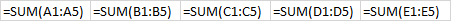Displaying them again in their R1C1 format, it is easy to see that the exact same formula is being used in all 5 cells: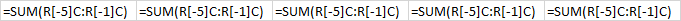The Inconsistent Formulas tools look for patterns where the R1C1 formulas do not match along a row or down a column.

### Find Inconsistent Formulas in Columns / Rows

Excel has a built-in Inconsistent Formula error check, but unfortunately it is not fool proof. The Find Inconsistent Formulas tool is a more robust method for checking the correctness of formulas in specified rows or columns. To use the tool, simply select the rows or columns to audit, click the button on the Spreadspeed ribbon, and the inconsistent formulas will be highlighted using the built-in Bad style.

#### Find Inconsistent Formulas Example

The example below highlights two of the issues that the built-in Inconsistent Formula error check in Excel will miss but Spreadspeed will properly identify. In cell B7, the =SUM() function has been replaced with a value. It's not a formula, so it's not inconsistent per the built-in error check. In cell D6, the formula has been modiified by multiplying a small fudge factor to the =AVERAGE(). The built-in inconsistent formula error check often will not flag an inconsistent formula in the last row or column of a series of formulas. The Spreadspeed Find Inconsistent Formulas tool does.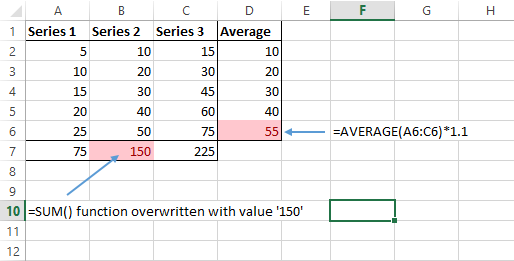### Flag Inconsistent Formulas in Worksheet

Because the Find Inconsistent Formulas tools described above operate on a selected range, they assume that the selected range should have the same R1C1 formula in every cell. Any deviations are marked as Bad. The Flag Inconsistent Formulas tool scans the entire worksheet, so it can't make the same assumption. Instead, it flags two types of inconsistencies:

• Explicit errors:
• The cells to the left and right (or above and below) have the same formula, but the cell in the middle does not.
• A cell with a value between two matching formulas.
• Pattern anomalies: Cells with formulas that match the cell to the left (or above), but not the cell to the right (or below).

#### Example: Multiple Formulas Altered

The first type of anomaly is when two or more formulas change in a row or column. In the image below, the middle cell has an altered formula, so Excel flags it with an error notification:However, if two cells have an altered formula, Excel will no longer flag them with the error notification: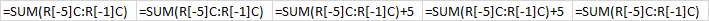Running the Flag Inconsistent Formulas tool, Spreadspeed will mark the cells in the region with the Check Cell style, as shown below, to indicate that there may be a problem in the region.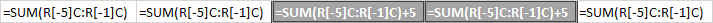#### Example: Formula in Last Column or Row

In the next example, we see another row of cells with no Excel error notification: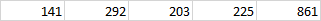Looking at the formulas, we see that the formula in the last column is different from the others. Excel usually won't flag these as inconsistent formula errors because it's common to have a sum, average, or other equation in the last column.Again, running the Flag Inconsistent Formulas tool will mark the cells in the region with the Check Cell style, as shown below. In this case, the different formula may be intentional, which is why Spreadspeed marks the region as Check Cell instead of marking it as Bad.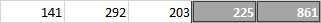(The tools on this page are just a small fraction of the Spreadspeed formula auditing toolset. The Precedents / Dependents Navigator, Visual Audit tools, and Audit Reports are also excellent for finding potential problems in both simplex calculations and complex models.)

Audit Reports | Formula Tools | Comment Tools